# Mechanics quiz

#### Quiz Description

In this quiz, we shall take a look at Mechanics, and a lot of exciting concepts like forces, velocity, dimensions, etc will be reviewed with the aid of the following questions. You will be guided through the journey of mastering the topic as you practice along.

Mechanics is one of the major branches of physics, and it is concerned with the motion of physical objects or bodies. To be more specific, mechanics studies the relationship between forces, motion, and matter. Forces are applied on bodies and cause them to either begin the motion, end motion, or change direction in motion. Notwithstanding, there are two branches of mechanics which are classical and quantum. Classical being concerned with the motion of bodies under the influence of a force or forces, and Quantum mechanics dealing with the explanation of the functioning of the universe at a scale smaller than atoms.

Are you looking forward to having a good pass in this section of Physics? All you need to do is take the quiz and improve on your knowledge. Good luck as you solve it!

A physical equation is dimensionally homogeneous when:

Correct
• It has good units
• The dimensions of the LHS=Dimensions of RHS
• The equation has no dimensionless quantities
• The equation can be divided using dimensions

The method of dimensions

Correct
• Doesn’t tell you when the equation is wrong
• Cannot be used to derive an equation
• Has nothing to do with the units
• None of the above

A graph cannot be used to

Correct
• Obtain values of some physical quantity
• Find the rate of change of none quantity with respect to another
• Predict the values of none quantity after some given time
• Checks the correctness of an equation.

The resultant of the two forces is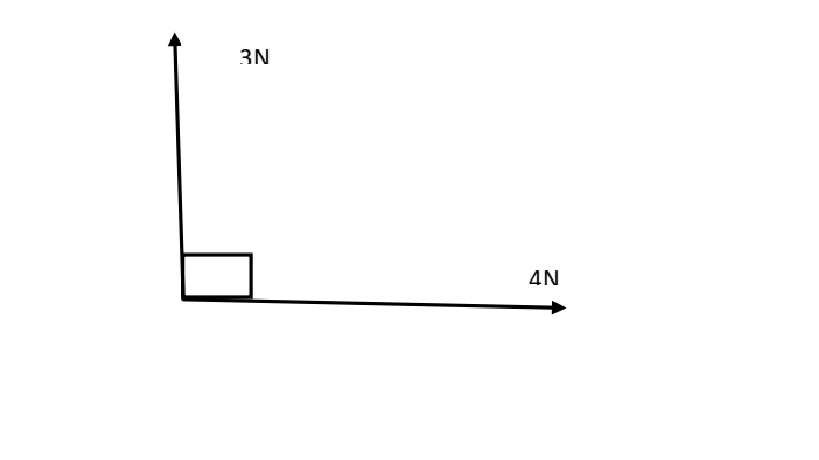Correct
• 1N
• 7N
• 5N
• 12N

The area under a force velocity graph gives

Correct
• Acceleration
• Momentum
• Work done
• Power

The graph presents the motion of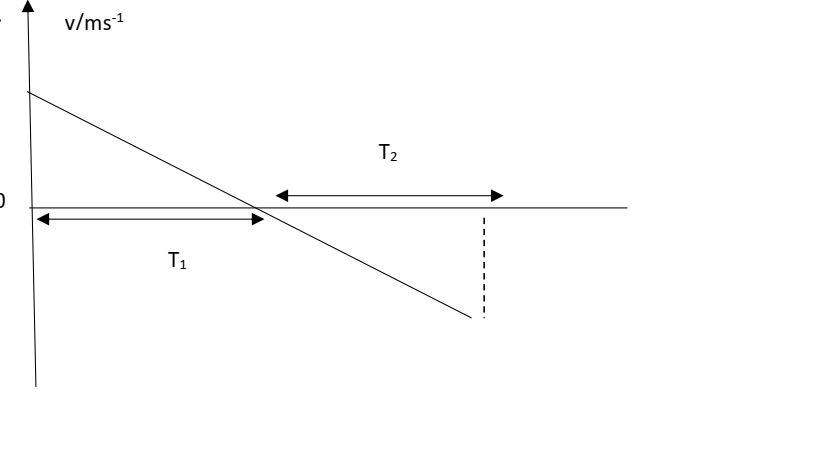Correct
• A body falling freely
• A body moving with a uniform acceleration
• A body falling freely from rest and going uniformly decelerating
• A body uniformly decelerating

The bob of the simple pendulum is set in oscillation. If strings cuts when the bob is passing through point B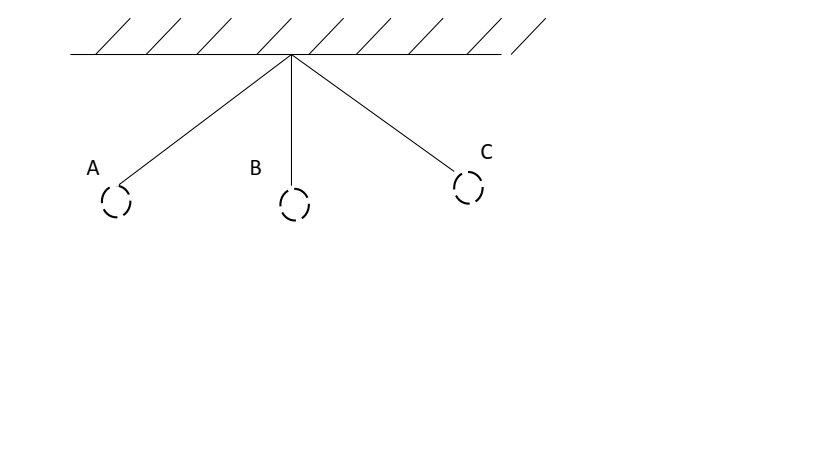Correct
• The bob will fall vertically from point B
• The bob will go beyond point C before falling
• The path described by the bob will be given by h= gx2/2u2 where h=height of the bob u=horizontal velocity of the bob from the ground of the box, x= horizontal distance travelled by bob.
• The bob will describe circular motion

The units Ns are the same as

Correct
• Kgm-1s-2
• Kgms-2
• Kgms2
• Kgm2s-2

When two bodies collide elastically

Correct
• They stick together and move off with one velocity
• Their energies increase considerably
• Momentum and kinetic energy are conserved
• The coefficient of restitution is less than one.

When a big body explodes into two unequal masses m1 and m2

Correct
• .
• .
• .
• P1 > p2 where P1 and P2 are the momenta of m1 and m2 respectively

The above graph represents the variations of energy of energy with time for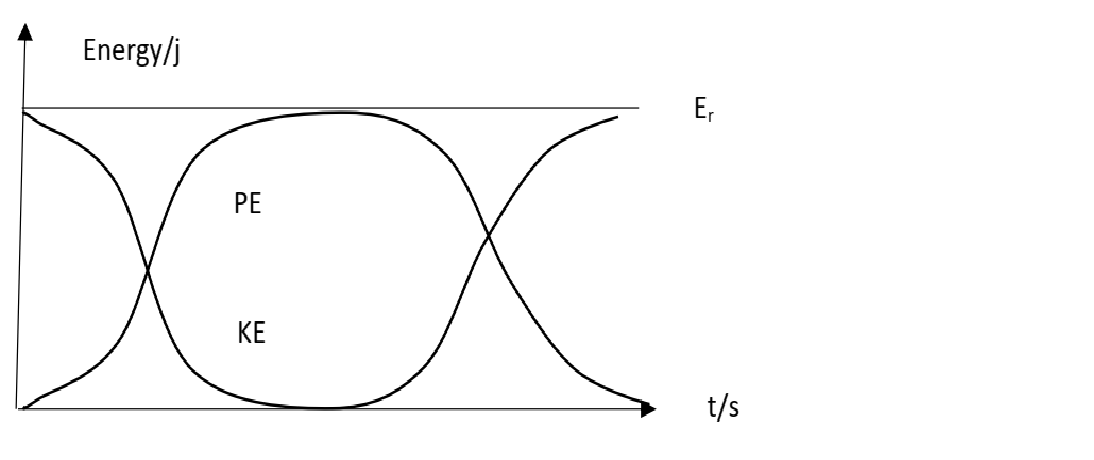Correct
• A body oscillating freely in air e.g simple pendulum
• A ball bounced once on the floor
• A simple pendulum oscillating in a windy area
• A ball in air and caught at the starting point

When the work done in moving round a closed path in a field to the original point is zero, the forces in
that field are called

Correct
• Contact forces
• Non-contact forces
• Strong forces
• Conservative forces

The forces F has a magnitude: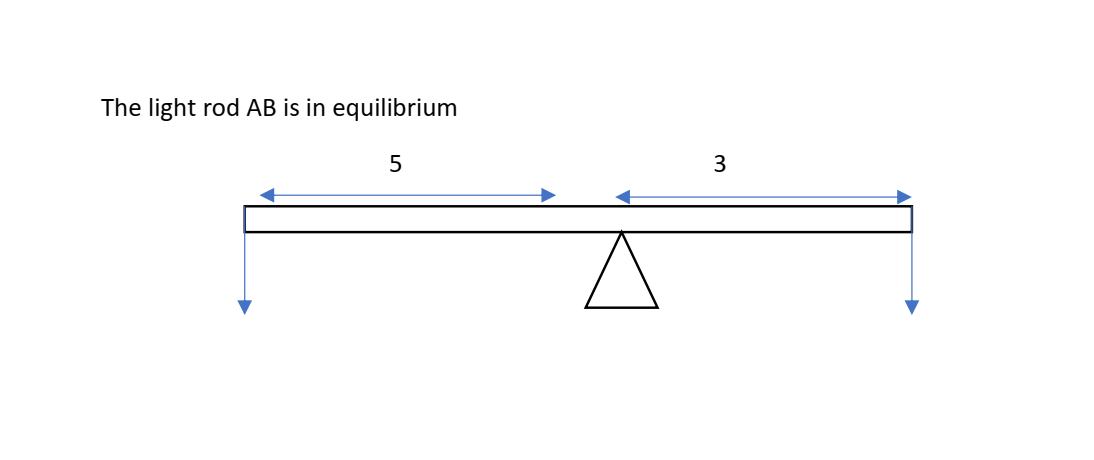Correct
• 36N
• 4N
• 10N
• 100N

A stone of mass 2kg is at the end of a string length 1m. if the stone is whirled round in a vertical circle at
4m. The Maximum tension in the string is

Correct
• 20N
• 13N
• 32N
• 44N

When a body of mass m moves in a circular path of radius r at a speed v.

Correct
• It accerates
• The centripetal force on it is given by Fc = 𝑚𝑣2/ 𝑟
• Its direction keeps changing
• All of the above